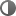## CI: Best Method to Estimate RMR (2010)

Citation:
Ireton-Jones C, Jones JD.  Improved equations for predicting energy expenditure in patients:  the Ireton-Jones equations.  Nutr Clin Pract 2002;17(1):29-31. PubMed ID: 16214963

Study Design:
Cross-Sectional Study
Class:
Quality Rating:NEUTRAL: See Quality Criteria Checklist below.
Research Purpose:
To further evaluate the original data from the development of the Ireton-Jones equations to determine if their precision could be enhanced and to compare the Harris-Benedict equations with measured energy expenditure to determine if a statistically significant correlation could be obtained.
Inclusion Criteria:
Ventilator-dependent and spontaneously breathing patients hospitalized in an acute care setting.  Patients stratified according to diagnosis:  burn, trauma or other (diabetes, pancreatitis, cancer).
Exclusion Criteria:
None specifically mentioned.
Description of Study Protocol:

Recruitment

Design

Cross-sectional study.

Blinding used (if applicable)

Not applicable.

Intervention (if applicable)

RMR measured with indirect calorimetry and estimated with equations.

Statistical Analysis

Multivariate regression analysis was used to correlate easily measurable patient data with measured energy expenditure using indirect calorimetry.  The 3 measures used to compare the previous equations and revised equations were the correlation coefficient between the equations and MEE, the mean of the prediction error between the equations and MEE, and the number of patients with MEE > the IJ equations vs number with MEE < IJ equations.  A comparative analysis was also made for the entire 300-patient population between Ireton-Jones and Harris-Benedict equations using actual body weight and total kcal/kg.

Data Collection Summary:

Timing of Measurements

RMR measured with indirect calorimetry and estimated using equations.

Dependent Variables

• RMR measured with indirect calorimetry, details not provided
• RMR estimated using Ireton-Jones equations
• RMR estimated using Harris-Benedict equations, multiplied by factor of 1.3

Independent Variables

• Height, weight
• Gender
• Age
• Diagnosis
• Ventilatory status
• Obesity

Control Variables

Description of Actual Data Sample:

Initial N: original equations developed using 200 patients, validated using second group of 100 patients

Attrition (final N):  300 patients from both groups

Age:  not mentioned

Ethnicity:  not mentioned

Other relevant demographics:  99 total ventilator dependent

Anthropometrics:

Location:  Texas

Summary of Results:

 r Value Mean prediction error (kcal/day) % of patients with MEE above predicted Original IJEE(v) 0.58 -271 35% Revised IJEE (v) 0.58 8 48% IJEE (actual body wt) 0.69 -6 48% HBEE (actual body wt) 0.60 410 76% HBEE x 1.3 (actual wt) 0.60 -51 41% 21 kcal/day 0.46 503 81%

Other Findings

Statistical analysis of the spontaneously breathing patients showed no improvement in predictability of energy expenditure, therefore, no change was made to this equation.

The statistical analysis for the ventilator-dependent patients demonstrated that the equations could be improved to minimize the error by adjusting numerical values in the equation.

The mean prediction error of the original equation was -271 kcal/day, while the revised equation showed a mean prediction error of 8 kcal/day, while still maintaining the strong correlation.

The Harris-Benedict equation, multiplied by 1.3, hads a reasonable correlation but consistently predicted energy expenditure lower than the MEE.

For obese patients, the Ireton-Jones equations with actual body weight was significantly correlated with MEE (r = 0.67) and the mean prediction error was -96 kcal/day.

Harris-Benedict x 1.3 calculated with actual body weight for obese patients significantly correlated to MEE (r = 0.64), but the mean prediction error was -177 kcal/day.

Author Conclusion:
The Ireton-Jones equations for both spontaneously breathing patients and the revised equation for ventilator-dependent patients minimize the error in predicting energy expenditure for both obese and nonobese patients.  For all patients, including obese patients, the high r value indicates low variability from the MEE, and the corresponding mean prediction error shows that this predictive equation will be closest to the actual MEE.
Funding Source:
 University/Hospital: Coram Healthcare